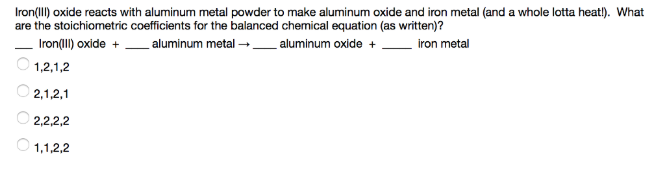# Problem: Iron(III) oxide reacts with aluminum metal powder to make aluminum oxide and iron metal (and a whole lotta heat!). What are the stoichiometric coefficients for the balanced chemical equation (as written)? _____ Iron (III) oxide + ____ aluminum metal → _____ aluminum oxide + _____ iron metal

###### FREE Expert Solution
99% (37 ratings)###### Problem Details

Iron(III) oxide reacts with aluminum metal powder to make aluminum oxide and iron metal (and a whole lotta heat!). What are the stoichiometric coefficients for the balanced chemical equation (as written)?

_____ Iron (III) oxide + ____ aluminum metal → _____ aluminum oxide + _____ iron metal